This mathematics ClipArt gallery offers 213 illustrations of common geometric constructions. Geometric constructions are made with only the use of a compass and a straight edge. In addition to the constructions of different types of polygons, images include those used to show how to bisect a line, angle, and arc.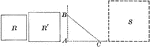### Square Constructed to be Equal to the Sum of Two Given Squares

Illustration of a how to construct a square equivalent to the sum of two given squares.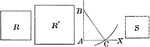### Square Constructed to be Equal to the Difference of Two Given Squares

Illustration of a how to construct a square equivalent to the difference of two given squares.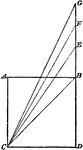### Construction of Multiple of a Square

Illustration used to construct a square that shall be a multiple of any given square.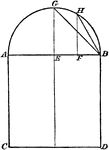### Construction of Proportional Square

Illustration used to construct a square that shall be in proportion to a given square.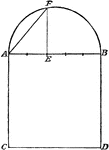### Construction of Proportional Square

Illustration used to construct a square that shall be in proportion to a given square.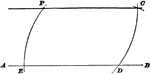### Parallel To Straight Line Construction

Illustration used to show how to draw a straight line parallel to a straight line through a given point.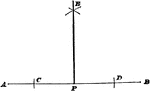### Perpendicular To Straight Line Construction

Illustration used to show how to draw a perpendicular to a straight line from a given point on the line…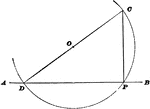### Perpendicular To Straight Line Construction

Illustration used to show how to draw a perpendicular to a straight line from a given point on the line…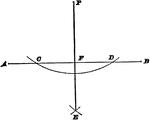### Perpendicular To Straight Line Construction

Illustration used to show how to draw a perpendicular to a straight line from a given point that is…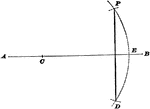### Perpendicular To Straight Line Construction

Illustration used to show how to draw a perpendicular to a straight line from a given point that is…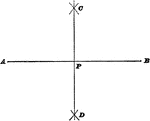### Bisect A Straight Line

Illustration used to show how to bisect a straight line.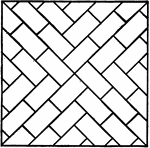### Drawing Street Paving Intersection

"An exercise in starting and stopping short lines. Draw three-inch square. Draw diagonals with 45-degree…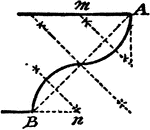### Construction Of A Talon

An illustration showing how to construct a talon, or two circle arcs that will tangent themselves, and…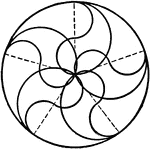### Drawing Tangent Arcs with Compass and Dividers

"Draw a circle three inches in diameter. Divide the circumference into five equal parts by trial with…### Construction Of Tangent Between 2 Circles

An illustration showing how to construct a tangent between 2 given circles. "Join the centers C and…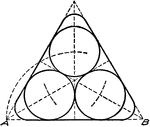### Drawing Tangent Circles and Lines with Compass and Triangles

"On base AB, 3 1/2" long construct an equilateral triangle, using the 60-degree triangle. Bisect the…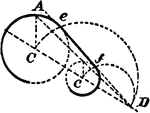### Construction Of Tangent To 2 Circles

An illustration showing how to construct a tangent to 2 given circles of different diameters. "Join…### Construction Of Circle Tangent To 2 Circles

An illustration showing how to construct a tangent circle to 2 given circles. "Join centers C and c…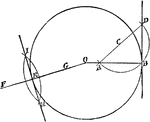### Construction of Tangent to a Circle

Illustration used to draw a tangent to a circle.### Construction of a Tangent to a Circle

Illustration used to show how "to construct a tangent to a circle from a point outside."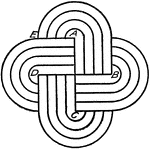### Drawing Tangent to Circle Arcs with Bow Compass

"Draw one and one-half inch square about center of space. Divide AE into four 3/16" spaces, with scale.…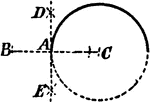### Construction Of Tangent To Circle

An illustration showing how to construct a tangent to a circle through a given point in a circumference.…### Construction Of Tangent To Circle

An illustration showing how to construct a tangent to a circle through a given point outside of a circumference.…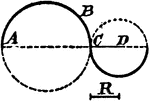### Construction Of Circle Tangent To Circle

An illustration showing how to construct a tangent circle to a circle with a given radius. "Through…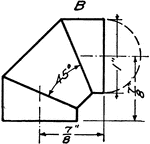### Development Exercise of Cylinder using Three Piece Elbow

The exercise problem of creating a three piece elbow development or rolled out image of the cylinder…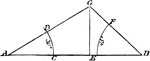### Construction of Triangle Given Angles and Base

Illustration used to construct a triangle given the length of the base and the two base angles.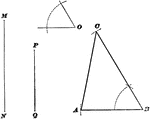### Construction Of Triangle

Illustration used to show how to draw a triangle when given two sides and the included angle of the…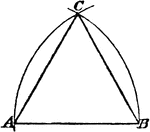### Construction of an Equilateral Triangle

Illustration used to construct an equilateral triangle on a given base.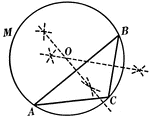### Construction Used to Circumscribe a Circle About a Triangle

Illustration used to circumscribe a circle about a given triangle.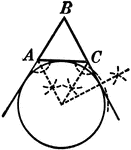### Construction Used to Escribe a Circle to a Triangle

Illustration used to escribe a circle to a given triangle. "A circle which is tangent to one side of…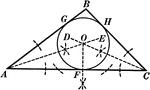### Construction Used to Inscribe a Circle in a Triangle

Illustration used to inscribe a circle in a given triangle.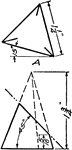### Development Exercise of Triangular Pyramid

Exercise problem to develop, or rolled out, image of the triangular pyramid by creating an equal length…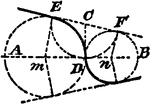### Construction Of Two Circles That Tangent Themselves and 2 Given Lines

An illustration showing how to construct two circles that tangent themselves and two given lines. "Draw…